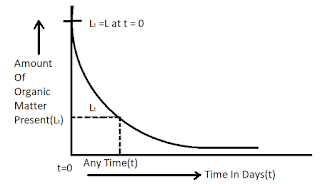Relation Between B.O.D (Biochemical Oxygen Demand) And Time - Civil Engineering

## Relation Between B.O.D (Biochemical Oxygen Demand) And Time

At certain temperature, the rate of deoxygenation is assumed to be directly proportional to the amount of organic matter present in the sewage at that time.
dLt / dt = - K.Lt .............(1)Relation Between B.O.D And Time

[Note: Minus sign indicates that the with the passage of time the value Lt of decreases]
Where, Lt = Organic matter present in sewage after t day from the start of oxidation in mg/lit.
t = Time in days , K = Rate constant.

Now find out the relation between B.O.D and time?
Integrating equation (1), we get
ഽdLt / Lt = ഽ- K. dt
or,
log Lt = - K.t + C ...........(2)
Where C is constant of integration .

When t = 0, Lt = L (say)
Now, substituting these in equation (2) we have,
log L = - K. (0) + C
Or, C = log L

Substituting this value of C in equation (2) we get
log Lt = -K.t + log L
Or, log (Lt/ L) = -K.t
Or, 2.303 log10 (Lt/ L) = -K.t
Or, log10 (Lt/ L) = - 0.434 K.t
Or, (Lt/ L) = (10)^(-0.434 k.t)

If Yt represents the total amount of organic matter oxidized in t days (i.e B.O.D), then we have
Yt = L- Lt = L [1- (Lt/ L)]
Or, Yt /L = L- Lt = 1- (Lt/ L)
Or, Yt /L = L- Lt = 1- (10)^(-0.434 k.t)
Or, Yt = L [1- (10)^(-0.434 k.t)